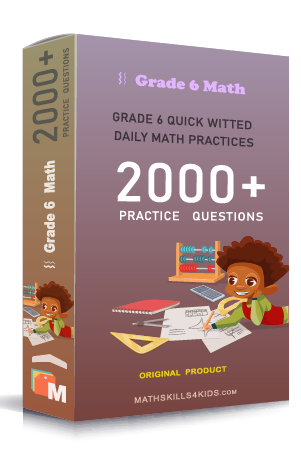# Grade 6 Multiplying fraction worksheets with solutions

Subject
Math

Resource Type
Worksheets, Printables, Homeschool

Format
PDF (22.3 MB | 32 pages → Exercises + Solutions)

• ### -15% OFF Over \$100

Promo Code: TOPLA

After Purchase

\$3.85

This package allows you to practice the following skills

Finding fractions of a whole number

Solving fractions of a whole number word problems

Estimating products of fractions and whole numbers

Multiplying two fractions using models

Finding the product of two fractions

Multiplying fractions word problems

Scaling whole numbers by fractions

Scaling by fractions and mixed numbers

Multiplying three or more fractions and whole numbers

Estimating products of fractions whole numbers and mixed numbers

Multiplying mixed numbers and whole numbers

Multiplying mixed numbers word problems

Multiplying three or more mixed numbers fractions and or whole numbers

This product is a part of the Mega Pack
* Grade 6 quick-witted daily math practices *